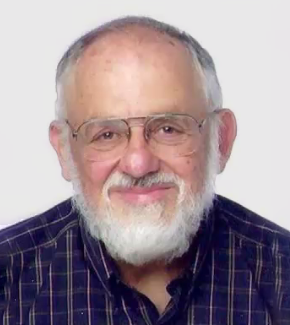# Learn Differential Equations

## Up close with Gilbert Strang and Cleve Moler

Gilbert Strang, professor and mathematician at Massachusetts Institute of Technology, and Cleve Moler, founder and chief mathematician at MathWorks, deliver an in-depth video series about differential equations and the MATLAB ODE suite. These videos are suitable for students and life-long learners to enjoy.### Differential Equations and Linear Algebra

Explore differential equations and the math formulas behind them with Professor Gilbert Strang.### Solving ODEs in MATLAB

Learn about the MATLAB ODE suite through examples and exercises with Cleve Moler.